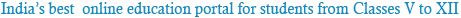### NCERT Solutions for Class 12th Chemistry Chapter 1: The Solid State

Question 1.1:
Define the term ‘amorphous’. Give a few examples of amorphous solids.
Amorphous solids are the solids whose constituent particles are of irregular shapes and have short range order. These solids are isotropic in nature and melt over a range of temperature. Therefore, amorphous solids are sometimes called pseudo solids or super cooled liquids. They do not have definite heat of fusion. When cut with a sharp-edged tool, they cut into two pieces with irregular surfaces. Examples of amorphous solid include glass, rubber, and plastic.

Question 1.2:
What makes a glass different from a solid such as quartz? Under what conditions could quartz be converted into glass?
The arrangement of the constituent particles makes glass different from quartz. In glass, the constituent particles have short range order, but in quartz, the constituent particles have both long range and short range orders.
Quartz can be converted into glass by heating and then cooling it rapidly.

Question 1.3:
Classify each of the following solids as ionic, metallic, molecular, network (covalent) or amorphous.
(i) Tetra phosphorus decoxide (P4O10) (vii) Graphite
(ii) Ammonium phosphate (NH4)3PO4 (viii) Brass
(iii) SiC (ix) Rb
(iv) I2 (x) LiBr
(v) P4 (xi) Si
Ionic → (ii) Ammonium phosphate (NH4)3PO4, (x) LiBr
Metallic → (viii) Brass, (ix) Rb
Molecular → (i) Tetra phosphorus decoxide (P4O10), (iv) I2, (v) P4.
Covalent (network) → (iii) SiC, (vii) Graphite, (xi) Si
Amorphous → (vi) Plastic

Question 1.4:
(i) What is meant by the term ‘coordination number’?
(ii) What is the coordination number of atoms:
(a) in a cubic close-packed structure?
(b) in a body-centred cubic structure?
(i) The number of nearest neighbours of any constituent particle present in the crystal lattice is called its coordination number.
(ii) The coordination number of atoms
(a) in a cubic close-packed structure is 12, and
(b) in a body-centred cubic structure is 8

Question 1.5:
How can you determine the atomic mass of an unknown metal if you know its density and the dimension of its unit cell? Explain.
By knowing the density of an unknown metal and the dimension of its unit cell, the atomic mass of the metal can be determined.
Let ‘a’ be the edge length of a unit cell of a crystal, ‘d’ be the density of the metal, ‘m’ be the atomic mass of the metal and ‘z’ be the number of atoms in the unit cell.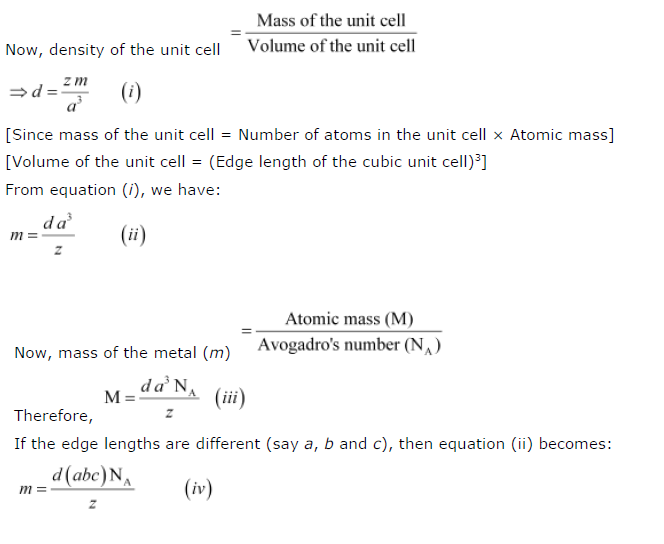From equations (iii) and (iv), we can determine the atomic mass of the unknown metal.

Question 1.6:
‘Stability of a crystal is reflected in the magnitude of its melting point’. Comment. Collect melting points of solid water, ethyl alcohol, diethyl ether and methane from a data book.
What can you say about the intermolecular forces between these molecules?
Higher the melting point, greater is the intermolecular force of attraction and greater is the stability. A substance with higher melting point is more stable than a substance with lower melting point.
The melting points of the given substances are:
Solid water → 273 K
Ethyl alcohol → 158.8 K
Diethyl ether → 156.85 K
Methane → 89.34 K
Now, on observing the values of the melting points, it can be said that among the given substances, the intermolecular force in solid water is the strongest and that in methane is the weakest.

Question 1.7:
How will you distinguish between the following pairs of terms:
(i) Hexagonal close-packing and cubic close-packing?
(ii) Crystal lattice and unit cell?
(iii) Tetrahedral void and octahedral void?
i.A 2-D hexagonal close-packing contains two types of triangular voids (a and b) as shown in figure 1. Let us call this 2-D structure as layer A. Now, particles are kept in the voids present in layer A (it can be easily observed from figures 2 and 3 that only one of the voids will be occupied in the process, i.e., either a or b). Let us call the particles or spheres present in the voids of layer A as layer B. Now, two types of voids are present in layer B (c and d). Unlike the voids present in layer A, the two types of voids present in layer B are not similar. Void c is surrounded by 4 spheres and is called the tetrahedral void. Void d is surrounded by 6 spheres and is called the octahedral void.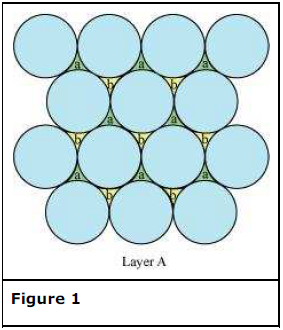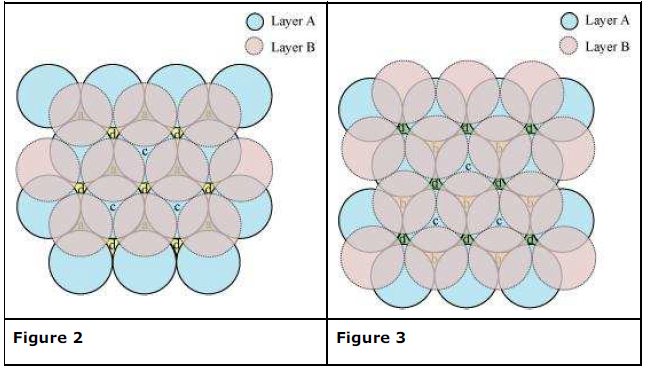Now, the next layer can be placed over layer B in 2 ways.
Case 1: When the third layer (layer C) is placed over the second one (layer B) in such a manner that the spheres of layer C occupy the tetrahedral voids c. In this case we get hexagonal close-packing. This is shown in figure 4. In figure 4.1, layer B is present over the voids a and layer C is present over the voids c. In figure 4.2, layer B is present over the voids b and layer C is present over the voids c. It can be observed from the figure that in this arrangement, the spheres present in layer C are present directly above the spheres of layer A. Hence, we can say that the layers in hexagonal close-packing are arranged in an ABAB….. pattern.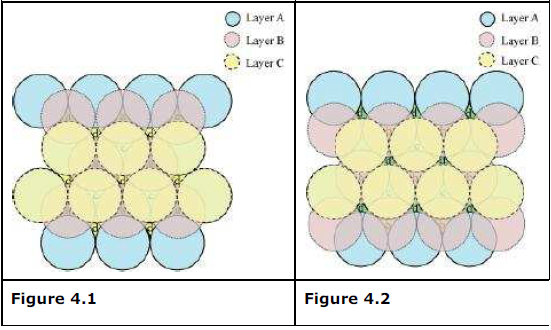Case 2: When the third layer (layer C) is placed over layer B in such a manner that the spheres of layer C occupy the octahedral voids d. In this case we get cubic close-packing. In figure 5.1, layer B is present over the voids a and layer C is present over the voids d. In figure 5.2, layer B is present over the voids b and layer C is present over the voids d. It can be observed from the figure that the arrangement of particles in layer C is completely different from that in layers A or B. When the fourth layer is kept over the third layer, the arrangement of particles in this layer is similar to that in layer A. Hence, we can say that the layers in cubic closepacking are arranged in an ABCABC….. pattern.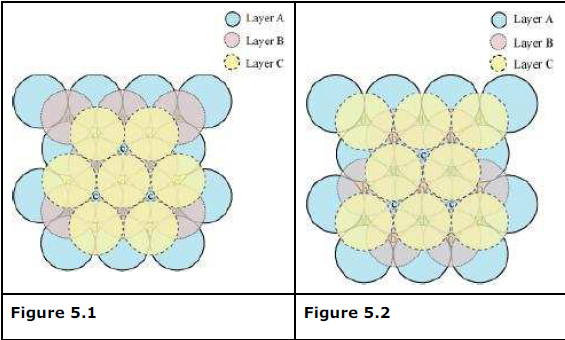The side views of hcp and ccp are given in figures 6.1 and 6.2 respectively.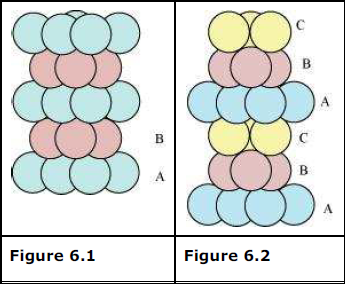(ii) The diagrammatic representation of the constituent particles (atoms, ions, or molecules) present in a crystal in a regular three-dimensional arrangement is called crystal lattice.
A unit cell is the smallest three-dimensional portion of a crystal lattice. When repeated again and again in different directions, it generates the entire crystal lattice.
(iii) A void surrounded by 4 spheres is called a tetrahedral void and a void surrounded by 6 spheres is called an octahedral void. Figure 1 represents a tetrahedral void and figure 2 represents an octahedral void.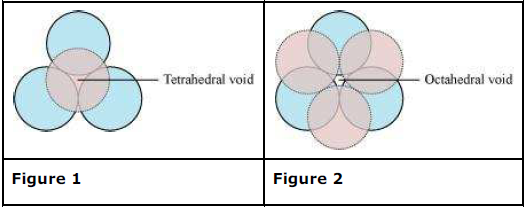Question 1.8:
How many lattice points are there in one unit cell of each of the following lattice?
(i) Face-centred cubic
(ii) Face-centred tetragonal
(iii) Body-centred
(i) There are 14 (8 from the corners + 6 from the faces) lattice points in face-centred cubic.
(ii) There are 14 (8 from the corners + 6 from the faces) lattice points in face-centred tetragonal.
(iii) There are 9 (1 from the centre + 8 from the corners) lattice points in body-centred cubic.

Question 1.9:
Explain
(i) The basis of similarities and differences between metallic and ionic crystals.
(ii) Ionic solids are hard and brittle.
(i) The basis of similarities between metallic and ionic crystals is that both these crystal types are held by the electrostatic force of attraction. In metallic crystals, the electrostatic force acts between the positive ions and the electrons. In ionic crystals, it acts between the oppositely-charged ions. Hence, both have high melting points.
The basis of differences between metallic and ionic crystals is that in metallic crystals, the electrons are free to move and so, metallic crystals can conduct electricity. However, in ionic crystals, the ions are not free to move. As a result, they cannot conduct electricity. However, in molten state or in aqueous solution, they do conduct electricity.
(ii) The constituent particles of ionic crystals are ions. These ions are held together in three-dimensional arrangements by the electrostatic force of attraction. Since the electrostatic force of attraction is very strong, the charged ions are held in fixed positions. This is the reason why ionic crystals are hard and brittle.

Question 1.10:
Calculate the efficiency of packing in case of a metal crystal for
(i) simple cubic
(ii) body-centred cubic
(iii) face-centred cubic (with the assumptions that atoms are touching each other).
(i) Simple cubic
In a simple cubic lattice, the particles are located only at the corners of the cube and touch each other along the edge.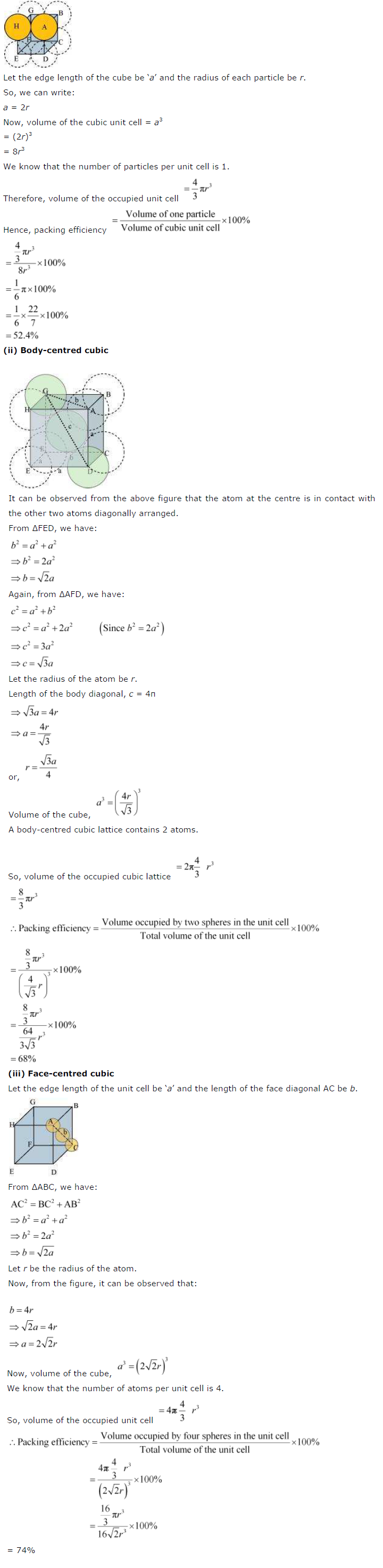Question 1.11:
Silver crystallises in fcc lattice. If edge length of the cell is 4.07 × 10−8 cm and density is 10.5 g cm−3, calculate the atomic mass of silver.
It is given that the edge length, a = 4.077 × 10−8 cm
Density, d = 10.5 g cm−3
As the lattice is fcc type, the number of atoms per unit cell, z = 4
We also know that, NA = 6.022 × 1023 mol−1
Using the relation: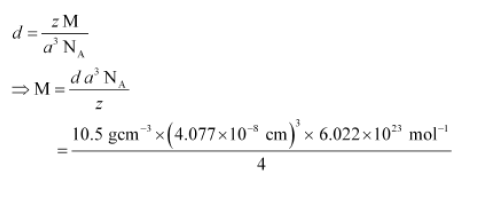= 107.13 gmol−1
Therefore, atomic mass of silver = 107.13 u

Question 1.12:
A cubic solid is made of two elements P and Q. Atoms of Q are at the corners of the cube and P at the body-centre. What is the formula of the compound? What are the coordination numbers of P and Q?
It is given that the atoms of Q are present at the corners of the cube.
Therefore, number of atoms of Q in one unit cell = 8 x (1/8) = 1
It is also given that the atoms of P are present at the body-centre.
Therefore, number of atoms of P in one unit cell = 1
This means that the ratio of the number of P atoms to the number of Q atoms, P:Q = 1:1
Hence, the formula of the compound is PQ.
The coordination number of both P and Q is 8.

Question 1.13:
Niobium crystallises in body-centred cubic structure. If density is 8.55 g cm−3, calculate atomic radius of niobium using its atomic mass 93 u.
It is given that the density of niobium, d = 8.55 g cm−3
Atomic mass, M = 93 gmol−1
As the lattice is bcc type, the number of atoms per unit cell, z = 2
We also know that, NA = 6.022 × 1023 mol^−1
Applying the relation: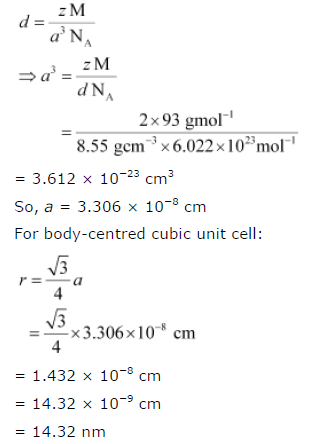Question 1.14:
If the radius of the octachedral void is r and radius of the atoms in close packing is R, derive relation between r and R.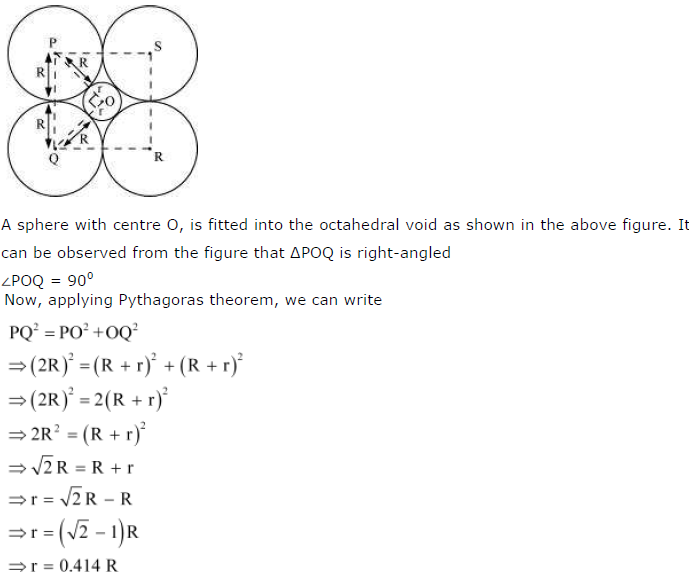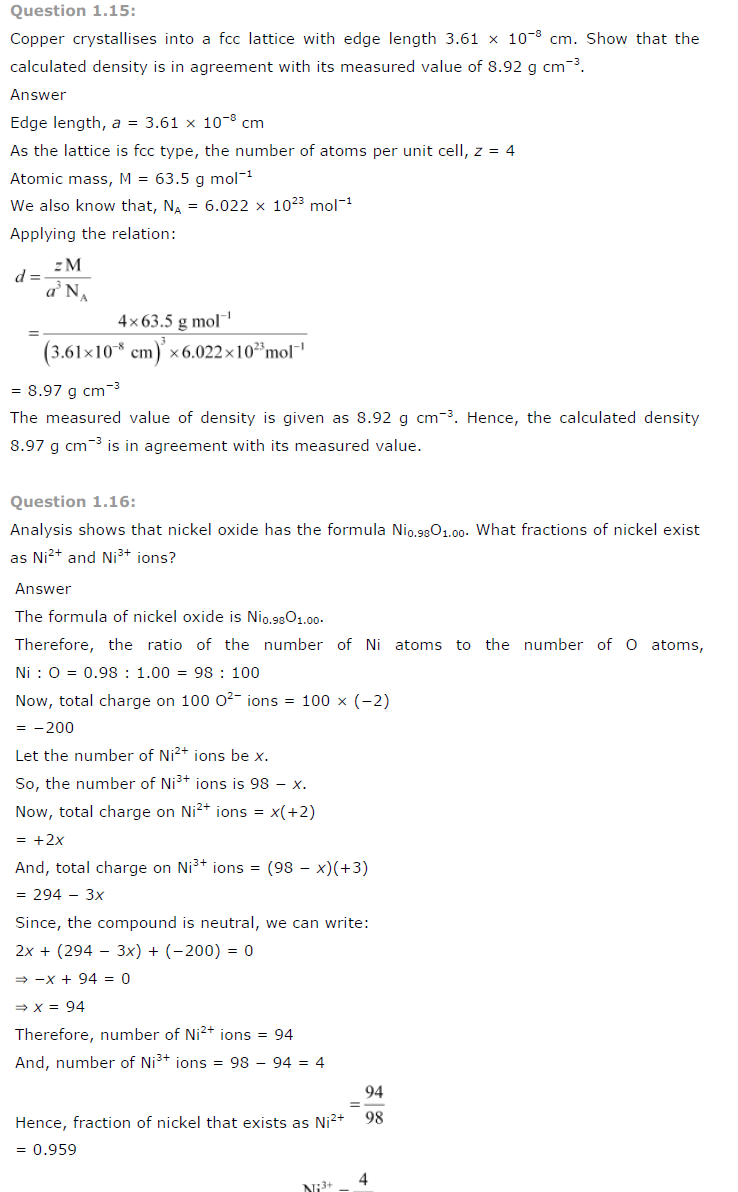Question 1.17:
What is a semiconductor? Describe the two main types of semiconductors and contrast their conduction mechanism.
Semiconductors are substances having conductance in the intermediate range of 10^-6 to 10^4 ohm^−1m^−1.
The two main types of semiconductors are:
(i) n-type semiconductor
(ii) p-type semiconductor
n-type semiconductor: The semiconductor whose increased conductivity is a result of negatively-charged electrons is called an n-type semiconductor. When the crystal of a group 14 element such as Si or Ge is doped with a group 15 element such as P or As, an n-type semiconductor is generated.
Si and Ge have four valence electrons each. In their crystals, each atom forms four covalent bonds. On the other hand, P and As contain five valence electrons each. When Si or Ge is doped with P or As, the latter occupies some of the lattice sites in the crystal. Four out of five electrons are used in the formation of four covalent bonds with four neighbouring Si or Ge atoms. The remaining fifth electron becomes delocalised and increases the conductivity of the doped Si or Ge.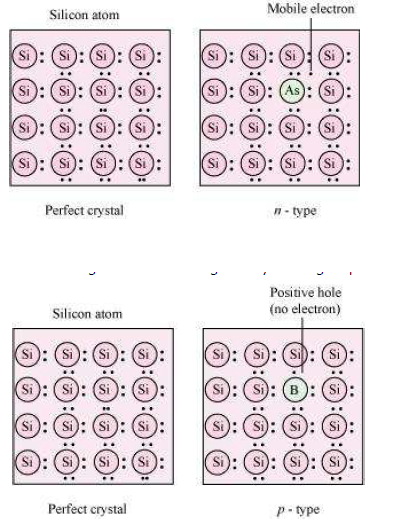p-type semiconductor: The semiconductor whose increased in conductivity is a result of electron hole is called a p-type semiconductor. When a crystal of group 14 elements such as Si or Ge is doped with a group 13 element such as B, Al, or Ga (which contains only three valence electrons), a p-type of semiconductor is generated.
When a crystal of Si is doped with B, the three electrons of B are used in the formation of three covalent bonds and an electron hole is created. An electron from the neighbouring atom can come and fill this electron hole, but in doing so, it would leave an electron hole at its original position. The process appears as if the electron hole has moved in the direction opposite to that of the electron that filled it. Therefore, when an electric field is applied, electrons will move toward the positively-charged plate through electron holes. However, it will appear as if the electron holes are positively-charged and are moving toward the negatively- charged plate.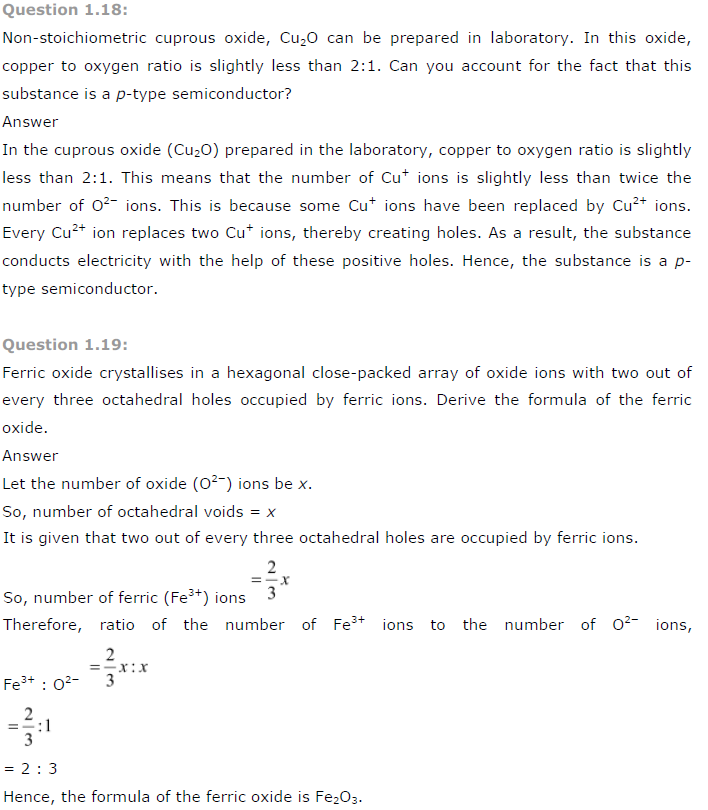Question 1.20:
Classify each of the following as being either a p-type or an n-type semiconductor:
(i) Ge doped with In (ii) B doped with Si.
(i) Ge (a group 14 element) is doped with In (a group 13 element). Therefore, a hole will be created and the semiconductor generated will be a p-type semiconductor.
(ii) B (a group 13 element) is doped with Si (a group 14 element). So, there will be an extra electron and the semiconductor generated will be an n-type semiconductor.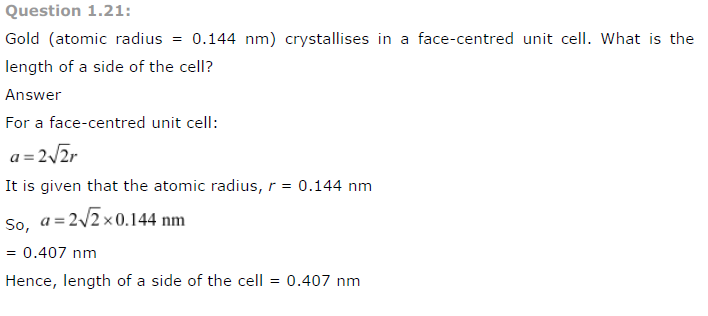Question 1.22:
In terms of band theory, what is the difference
(i) Between a conductor and an insulator
(ii) Between a conductor and a semiconductor
(i) The valence band of a conductor is partially-filled or it overlaps with a higher energy, unoccupied conduction band.
On the other hand, in the case of an insulator, the valence band is fully- filled and there is a large gap between the valence band and the conduction band.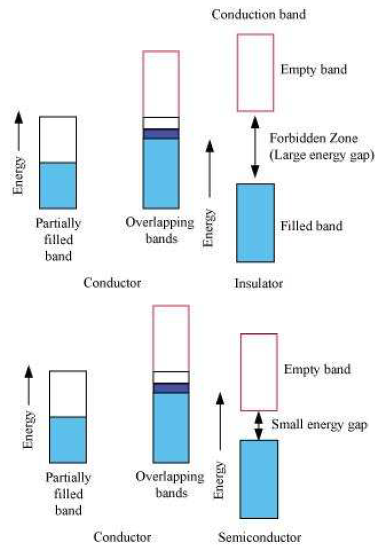(ii) In the case of a conductor, the valence band is partially-filled or it overlaps with a higher energy, unoccupied conduction band. So, the electrons can flow easily under an applied electric field.
On the other hand, the valence band of a semiconductor is filled and there is a small gap between the valence band and the next higher conduction band. Therefore, some electrons can jump from the valence band to the conduction band and conduct electricity.

Question 1.23:
Explain the following terms with suitable examples:
(i) Schottky defect
(ii) Frenkel defect
(iii) Interstitials and
(iv) F-centres
(i) Schottky defect: Schottky defect is basically a vacancy defect shown by ionic solids. In this defect, an equal number of cations and anions are missing to maintain electrical neutrality. It decreases the density of a substance. Significant number of Schottky defects is present in ionic solids. For example, in NaCl, there are approximately 106 Schottky pairs per cm3 at room temperature. Ionic substances containing similarsized cations and anions show this type of defect. For example: NaCl, KCl, CsCl, AgBr, etc.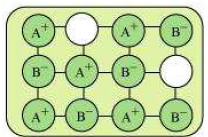(ii) Frenkel defect: Ionic solids containing large differences in the sizes of ions show this type of defect. When the smaller ion (usually cation) is dislocated from its normal site to an interstitial site, Frenkel defect is created. It creates a vacancy defect as well as an interstitial defect. Frenkel defect is also known as dislocation defect. Ionic solids such as AgCl, AgBr, AgI, and ZnS show this type of defect.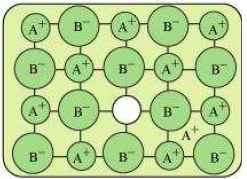(iii) Interstitials: Interstitial defect is shown by non-ionic solids. This type of defect is created when some constituent particles (atoms or molecules) occupy an interstitial site of the crystal. The density of a substance increases because of this defect.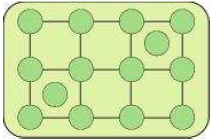(iv) F-centres: When the anionic sites of a crystal are occupied by unpaired electrons, the ionic sites are called F-centres. These unpaired electrons impart colour to the crystals. For example, when crystals of NaCl are heated in an atmosphere of sodium vapour, the sodium atoms are deposited on the surface of the crystal. The Cl ions diffuse from the crystal to its surface and combine with Na atoms, forming NaCl. During this process, the Na atoms on the surface of the crystal lose electrons. These released electrons diffuse into the crystal and occupy the vacant anionic sites, creating F-centres.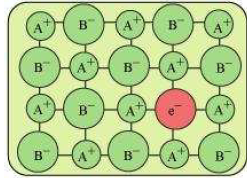Question 1.24:
Aluminium crystallises in a cubic close-packed structure. Its metallic radius is 125 pm.
(i) What is the length of the side of the unit cell?
(ii) How many unit cells are there in 1.00 cm3 of aluminium?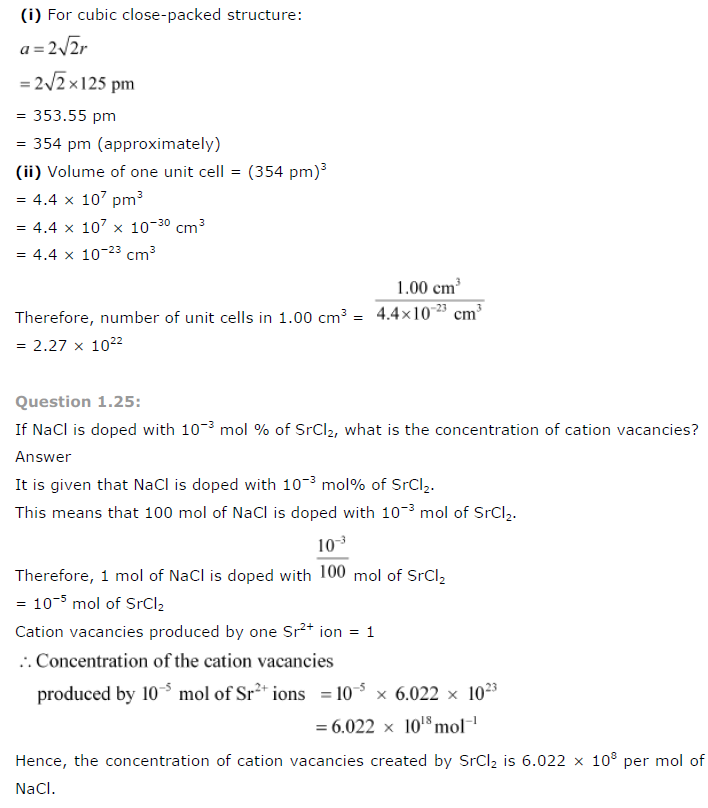Question 1.26:
Explain the following with suitable examples:
(i) Ferromagnetism
(ii)Paramagnetism
(iii)Ferrimagnetism
(iv)Antiferromagnetism
(v)12-16 and 13-15 group compounds.
(i) Ferromagnetism: The substances that are strongly attracted by a magnetic field are called ferromagnetic substances. Ferromagnetic substances can be permanently magnetised even in the absence of a magnetic field. Some examples of ferromagnetic substances are iron, cobalt, nickel, gadolinium, and CrO2. In solid state, the metal ions of ferromagnetic substances are grouped together into small regions called domains and each domain acts as a tiny magnet. In an unmagnetised piece of a ferromagnetic substance, the domains are randomly-oriented and so, their magnetic moments get cancelled. However, when the substance is placed in a magnetic field, all the domains get oriented in the direction of the magnetic field. As a result, a strong magnetic effect is produced. This ordering of domains persists even after the removal of the magnetic field. Thus, the ferromagnetic substance becomes a permanent magnet.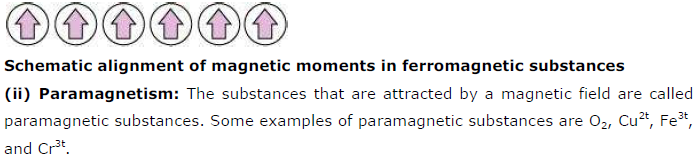Paramagnetic substances get magnetised in a magnetic field in the same direction, but lose magnetism when the magnetic field is removed. To undergo paramagnetism, a substance must have one or more unpaired electrons. This is because the unpaired electrons are attracted by a magnetic field, thereby causing paramagnetism.
(iii) Ferrimagnetism: The substances in which the magnetic moments of the domains are aligned in parallel and anti-parallel directions, in unequal numbers, are said to have ferrimagnetism. Examples include Fe3O4 (magnetite), ferrites such as MgFe2O4 and ZnFe2O4.
Ferrimagnetic substances are weakly attracted by a magnetic field as compared to ferromagnetic substances. On heating, these substances become paramagnetic.

(v) 12-16 and 13-15 group compounds: The 12-16 group compounds are prepared by combining group 12 and group 16 elements and the 13-15 group compounds are prepared by combining group 13 and group15 elements. These compounds are prepared to stimulate average valence of four as in Ge or Si. Indium (III) antimonide (IrSb), aluminium phosphide (AlP), and gallium arsenide (GaAS) are typical compounds of groups 13-15. GaAs semiconductors have a very fast response time and have revolutionised the designing of semiconductor devices. Examples of group 12-16 compounds include zinc sulphide (ZnS), cadmium sulphide (CdS), cadmium selenide (CdSe), and mercury (II) telluride (HgTe). The bonds in these compounds are not perfectly covalent. The ionic character of the bonds depends on the electronegativities of the two elements.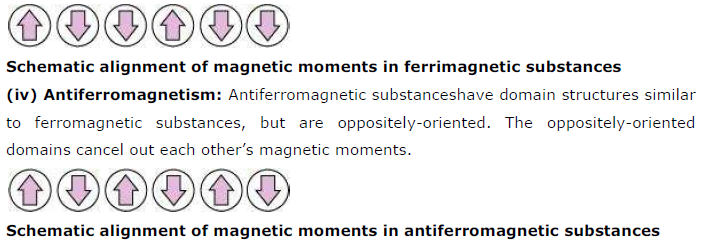Question 1.1:
Why are solids rigid?
The intermolecular forces of attraction that are present in solids are very strong. The constituent particles of solids cannot move from their positions i.e., they have fixed positions. However, they can oscillate about their mean positions. This is the reason solids are rigid.

Question 1.2:
Why do solids have a definite volume?
The intermolecular forces of attraction that are present in solids are very strong. The constituent particles of solids have fixed positions i.e., they are rigid. Hence, solids have a definite volume.

Question 1.3:
Classify the following as amorphous or crystalline solids:
Polyurethane, naphthalene, benzoic acid, teflon, potassium nitrate, cellophane, polyvinyl
chloride, fibre glass, copper.
Amorphous solids
Polyurethane, teflon, cellophane, polyvinyl chloride, fibre glass
Crystalline solids
Naphthalene, benzoic acid, potassium nitrate, copper

Question 1.4:
Why is glass considered a super cooled liquid?
Similar to liquids, glass has a tendency to flow, though very slowly. Therefore, glass is considered as a super cooled liquid. This is the reason that glass windows and doors are slightly thicker at the bottom than at the top.

Question 1.5:
Refractive index of a solid is observed to have the same value along all directions. Comment on the nature of this solid. Would it show cleavage property?
An isotropic solid has the same value of physical properties when measured along different directions. Therefore, the given solid, having the same value of refractive index along all directions, is isotropic in nature. Hence, the solid is an amorphous solid. When an amorphous solid is cut with a sharp edged tool, it cuts into two pieces with irregular surfaces.

Question 1.6:
Classify the following solids in different categories based on the nature of intermolecular forces operating in them:
Potassium sulphate, tin, benzene, urea, ammonia, water, zinc sulphide, graphite, rubidium, argon, silicon carbide.
Potassium sulphate → Ionic solid
Tin → Metallic solid
Benzene → Molecular (non-polar) solid
Urea → Polar molecular solid
Ammonia → Polar molecular solid
Water → Hydrogen bonded molecular solid
Zinc sulphide → Ionic solid
Graphite → Covalent or network solid
Rubidium → Metallic solid
Argon → Non-polar molecular solid
Silicon carbide → Covalent or network solid

Question 1.7:
Solid A is a very hard electrical insulator in solid as well as in molten state and melts at
extremely high temperature. What type of solid is it?
The given properties are the properties of a covalent or network solid. Therefore, the given solid is a covalent or network solid. Examples of such solids include diamond (C) and quartz (SiO2).

Question 1.8:
Ionic solids conduct electricity in molten state but not in solid state. Explain.
In ionic compounds, electricity is conducted by ions. In solid state, ions are held together by strong electrostatic forces and are not free to move about within the solid. Hence, ionic solids do not conduct electricity in solid state. However, in molten state or in solution form, the ions are free to move and can conduct electricity.

Question 1.9:
What type of solids are electrical conductors, malleable and ductile?
Metallic solids are electrical conductors, malleable, and ductile.

Question 1.10:
Give the significance of a ‘lattice point’.
The significance of a lattice point is that each lattice point represents one constituent particle of a solid which may be an atom, a molecule (group of atom), or an ion.

Question 1.11:
Name the parameters that characterize a unit cell.
The six parameters that characterise a unit cell are as follows.
(i) Its dimensions along the three edges, a, b, and c
These edges may or may not be equal.
(ii) Angles between the edges
These are the angle ∝ (between edges b and c), β (between edges a and c), and γ (between edges a and b).

Question 1.12:
Distinguish between
(i)Hexagonal and monoclinic unit cells
(ii) Face-centred and end-centred unit cells.
(i) Hexagonal unit cell
For a hexagonal unit cell,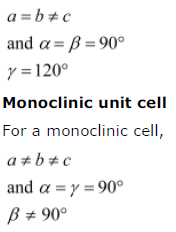(ii) Face-centred unit cell
In a face-centred unit cell, the constituent particles are present at the corners and one at the centre of each face.
End-centred unit cell
An end-centred unit cell contains particles at the corners and one at the centre of any two opposite faces.

Question 1.13:
Explain how much portion of an atom located at (i) corner and (ii) body-centre of a cubic unit cell is part of its neighbouring unit cell.
(i)An atom located at the corner of a cubic unit cell is shared by eight adjacent unit cells.
Therefore, 1/8 th portion of the atom is shared by one unit cell.
(ii)An atom located at the body centre of a cubic unit cell is not shared by its neighbouring unit cell. Therefore, the atom belongs only to the unit cell in which it is present i.e., its contribution to the unit cell is 1.

Question 1.14:
What is the two dimensional coordination number of a molecule in square close packed layer?
In square close-packed layer, a molecule is in contact with four of its neighbours.
Therefore, the two-dimensional coordination number of a molecule in square closepacked layer is 4.

Question 1.15:
A compound forms hexagonal close-packed structure. What is the total number of voids in 0.5 mol of it? How many of these are tetrahedral voids?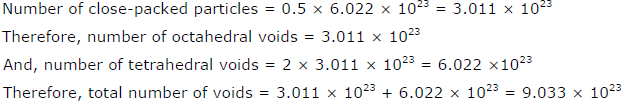Question 1.16:
A compound is formed by two elements M and N. The element N forms ccp and atoms of M occupy 1/3rd of tetrahedral voids. What is the formula of the compound?
The ccp lattice is formed by the atoms of the element N.
Here, the number of tetrahedral voids generated is equal to twice the number of atoms of the element N.
According to the question, the atoms of element M occupy 1/3rd of the tetrahedral voids.
Therefore, the number of atoms of M is equal to 2 x 1/3 = 2/3rd of the number of atoms of N.
Therefore, ratio of the number of atoms of M to that of N is M: N = (2/3) : 1 = 2:3
Thus, the formula of the compound is M2 N3.

Question 1.17:
Which of the following lattices has the highest packing efficiency (i) simple cubic (ii) body-centred cubic and (iii) hexagonal close-packed lattice?
Hexagonal close-packed lattice has the highest packing efficiency of 74%. The packing efficiencies of simple cubic and body-centred cubic lattices are 52.4% and 68% respectively.

Question 1.18:
An element with molar mass 2.7 × 10-2 kg mol-1 forms a cubic unit cell with edge length 405 pm. If its density is 2.7 × 103 kg m−3, what is the nature of the cubic unit cell?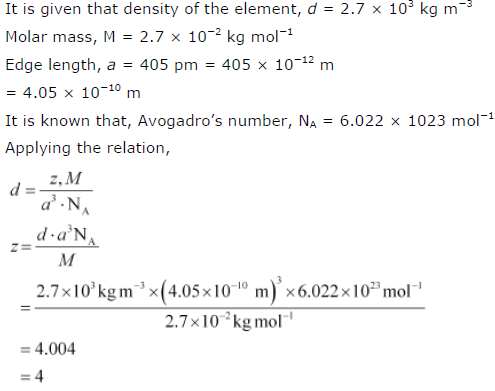This implies that four atoms of the element are present per unit cell. Hence, the unit cell is face-centred cubic (fcc) or cubic close-packed (ccp).

Question 1.19:
What type of defect can arise when a solid is heated? Which physical property is affected by it and in what way?
When a solid is heated, vacancy defect can arise. A solid crystal is said to have vacancy defect when some of the lattice sites are vacant.
Vacancy defect leads to a decrease in the density of the solid.

Question 1.20:
What type of stoichiometric defect is shown by:
(i) ZnS (ii) AgBr
(i) ZnS shows Frenkel defect.
(ii) AgBr shows Frenkel defect as well as Schottky defect.

Question 1.21:
Explain how vacancies are introduced in an ionic solid when a cation of higher valence is added as an impurity in it.
When a cation of higher valence is added to an ionic solid as an impurity to it, the cation of higher valence replaces more than one cation of lower valence so as to keep the crystal electrically neutral. As a result, some sites become vacant. For example, when Sr2+ is added to NaCl, each Sr2+ ion replaces two Na+ ions. However, one Sr2+ ion occupies the site of one Na+ ion and the other site remains vacant. Hence, vacancies are introduced.

Question 1.22:
Ionic solids, which have anionic vacancies due to metal excess defect, develop colour. Explain with the help of a suitable example.
The colour develops because of the presence of electrons in the anionic sites. These electrons absorb energy from the visible part of radiation and get excited. For example, when crystals of NaCl are heated in an atmosphere of sodium vapours, the sodium atoms get deposited on the surface of the crystal and the chloride ions from the crystal diffuse to the surface to form NaCl with the deposited Na atoms. During this process, the Na atoms on the surface lose electrons to form Na+ ions and the released electrons diffuse into the crystal to occupy the vacant anionic sites. These electrons get excited by absorbing energy from the visible light and impart yellow colour to the crystals.

Question 1.23:
A group 14 element is to be converted into n-type semiconductor by doping it with a suitable impurity. To which group should this impurity belong?
An n-type semiconductor conducts because of the presence of extra electrons. Therefore, a group 14 element can be converted to n-type semiconductor by doping it with a group 15 element.

Question 1.24:
What type of substances would make better permanent magnets, ferromagnetic or ferrimagnetic. Justify your answer.Powered by: iVerticals Web Technology Pvt Ltd